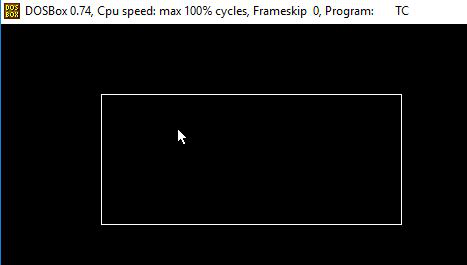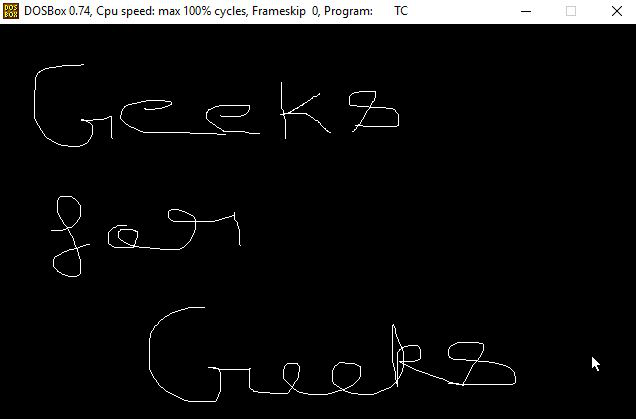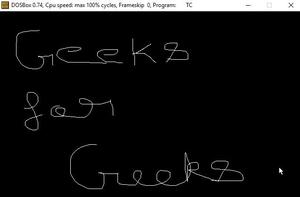# Use cases of mouse programming in C/C++ | Set 2

• Difficulty Level : Expert
• Last Updated : 28 Nov, 2021

In this article, we will discuss some use cases of Mouse Programming:

### Restrict mouse pointer:

The mouse pointer can be restricted in particular rectangle. The idea is to create a function called restrictmouse() which takes four parameters which containing X coordinate and Y coordinate. First point mention the top of the rectangle and the second point mention the bottom of the rectangle. Below are the functions used for the same:

• initmouse(): use to initialize mouse.
• showmouse(): shows the mouse pointer on the output screen.
• restrictmouse(): used to set Horizontal and vertical limit of the mouse pointer by setting the following parameters. AX = 7 for horizontal and AX = 8 for vertical.

Below is the program for the same:

## C

 `// C program to restrict the mouse``// pointer``#include ``#include ``#include ``#include ``union` `REGS in, out;`` ` `// Function to initialize the mouse``// pointer using graphics``int` `initMouse()``{``    ``in.x.ax = 0;``    ``int86(0X33, &in, &out);`` ` `    ``return` `out.x.ax;``}`` ` `// Function to display the mouse``// pointer using graphics``void` `showMouse()``{``    ``in.x.ax = 1;``    ``int86(0X33, &in, &out);``}`` ` `// Function to restrict the mouse``// pointers``void` `restrictMouse(``int` `x1, ``int` `y1,``                   ``int` `x2, ``int` `y2)``{``    ``// Set Horizontal limit``    ``in.x.ax = 7;``    ``in.x.cx = x1;``    ``in.x.dx = x2;``    ``int86(0X33, &in, &out);`` ` `    ``// Set Vertical limit``    ``in.x.ax = 8;``    ``in.x.cx = y1;``    ``in.x.dx = y2;``    ``int86(0X33, &in, &out);``}`` ` `// Driver Code``void` `main()``{``    ``int` `status, i, gd = DETECT, gm;`` ` `    ``// Initialize graphics``    ``initgraph(&gd, &gm, ``"C:\\TURBOC3\\BGI"``);`` ` `    ``// Get the status of the mouse``    ``status = initMouse();`` ` `    ``// Check if mouse is available or not``    ``if` `(status == 0)``        ``printf``(``"Mouse support "``               ``"not available.\n"``);``    ``else` `{``        ``showMouse();`` ` `        ``// Draw rectangle for displaying``        ``// the boundary``        ``rectangle(100, 70, 400, 200);``        ``restrictMouse(100, 70, 400, 200);``    ``}``    ``getch();`` ` `    ``// Close the graphics``    ``closegraph();`` ` `    ``return` `0;``}`

## C++

 `// C++ program to restrict the mouse``// pointer``#include ``#include ``#include ``#include ``#include ``using` `namespace` `std;`` ` `union` `REGS in, out;`` ` `// Function to initialize the mouse``// pointer using graphics``int` `initMouse()``{``    ``in.x.ax = 0;``    ``int86(0X33, &in, &out);`` ` `    ``return` `out.x.ax;``}`` ` `// Function to display the mouse``// pointer using graphics``void` `showMouse()``{``    ``in.x.ax = 1;``    ``int86(0X33, &in, &out);``}`` ` `// Function to restrict the mouse``// pointers``void` `restrictMouse(``int` `x1, ``int` `y1,``                   ``int` `x2, ``int` `y2)``{``    ``// Set Horizontal limit``    ``in.x.ax = 7;``    ``in.x.cx = x1;``    ``in.x.dx = x2;``    ``int86(0X33, &in, &out);`` ` `    ``// Set Vertical limit``    ``in.x.ax = 8;``    ``in.x.cx = y1;``    ``in.x.dx = y2;``    ``int86(0X33, &in, &out);``}`` ` `// Driver Code``void` `main()``{``    ``int` `status, i, gd = DETECT, gm;`` ` `    ``// Initialize graphics``    ``initgraph(&gd, &gm, ``"C:\\TURBOC3\\BGI"``);`` ` `    ``// Get the status of the mouse``    ``status = initMouse();`` ` `    ``// Check if mouse is available or not``    ``if` `(status == 0)``        ``cout << ``"Mouse support "``             ``<< ``"not available.\n"``;``    ``else` `{``        ``showMouse();`` ` `        ``// Draw rectangle for displaying``        ``// the boundary``        ``rectangle(100, 70, 400, 200);``        ``restrictMouse(100, 70, 400, 200);``    ``}``    ``getch();`` ` `    ``// Close the graphics``    ``closegraph();`` ` `    ``return` `0;``}`

Output:### Free hand drawing:

This following program makes use of some sub function, which were already discussed previously, and shows how they can be used to write useful programs like free-hand drawing. Below are the functions used:

• initmouse(): use to initialize mouse.
• showmouse(): shows mouse pointer on the output screen.
• hidemouse(): used to hide mouse while drawing.
• getmouseposition(): Fetches current location of the pointer and draw line accordingly.

Below is the program for the same:

## C

 `// C program to perform Free Hand Drawing``// using mouse programming``#include ``#include ``#include ``union` `REGS in, out;`` ` `// Function to initialize the mouse``// pointer using graphics``int` `initMouse()``{``    ``in.x.ax = 0;``    ``int86(0X33, &in, &out);`` ` `    ``return` `out.x.ax;``}`` ` `// Function to display the mouse``// pointer using graphics``void` `showMouse()``{``    ``in.x.ax = 1;``    ``int86(0X33, &in, &out);``}`` ` `// Function to hide the mouse``// pointer using graphics``void` `hideMouse()``{``    ``// Set AX=2 to hide mouse``    ``in.x.ax = 2;``    ``int86(0X33, &in, &out);``}`` ` `// Function to get the exact position``// of the mouse``getMousePosition(``int``* x, ``int``* y,``                 ``int``* click)``{``    ``in.x.ax = 3;`` ` `    ``// Get the coordinates``    ``int86(0x33, &in, &out);`` ` `    ``// Update the coordinates``    ``*x = out.x.cx;``    ``*y = out.x.dx;``    ``*click = out.x.bx & 1;``}`` ` `// Driver Code``void` `main()``{``    ``int` `status, i, gd = DETECT, gm,x1,y1,x2,y2;`` ` `    ``// Initialize graphics``    ``initgraph(&gd, &gm, ``"C:\\TURBOC3\\BGI"``);`` ` `    ``initMouse();`` ` `    ``// kbhit If a key has been pressed``    ``// then it returns a non zero value``    ``// otherwise returns zero(false)``    ``while` `(!kbhit()) {`` ` `        ``// Show the mouse pointer``        ``showMouse();`` ` `        ``// Get the mouse position``        ``getMousePosition(&x1, &y1, &click);``        ``x2 = x1;``        ``y2 = y1;`` ` `        ``// When mouse is clicked``        ``while` `(click == 1) {``            ``hideMouse();`` ` `            ``// Draw line``            ``line(x1, y1, x2, y2);``            ``x1 = x2;``            ``y1 = y2;`` ` `            ``// Get the mouse position``            ``getMousePosition(&x2, &y2, &click);``        ``}``    ``}`` ` `    ``getch();`` ` `    ``// Close the graphics``    ``closegraph();`` ` `    ``return` `0;``}`

## C++

 `// C++ program to perform Free Hand``// Drawing using mouse programming``#include ``#include ``#include ``#include ``using` `namespace` `std;`` ` `union` `REGS in, out;`` ` `// Function to initialize the mouse``// pointer using graphics``int` `initMouse()``{``    ``in.x.ax = 0;``    ``int86(0X33, &in, &out);`` ` `    ``return` `out.x.ax;``}`` ` `// Function to display the mouse``// pointer using graphics``void` `showMouse()``{``    ``in.x.ax = 1;``    ``int86(0X33, &in, &out);``}`` ` `// Function to hide the mouse``// pointer using graphics``void` `hideMouse()``{``    ``// Set AX=2 to hide mouse``    ``in.x.ax = 2;``    ``int86(0X33, &in, &out);``}`` ` `// Function to get the exact position``// of the mouse``getMousePosition(``int``* x, ``int``* y,``                 ``int``* click)``{``    ``in.x.ax = 3;`` ` `    ``// Get the coordinates``    ``int86(0x33, &in, &out);`` ` `    ``// Update the coordinates``    ``*x = out.x.cx;``    ``*y = out.x.dx;``    ``*click = out.x.bx & 1;``}`` ` `// Driver Code``void` `main()``{``    ``int` `status, i, gd = DETECT, gm;`` ` `    ``// Initialize graphics``    ``initgraph(&gd, &gm, ``"C:\\TURBOC3\\BGI"``);`` ` `    ``initMouse();`` ` `    ``// kbhit If a key has been pressed``    ``// then it returns a non zero value``    ``// otherwise returns zero(false)``    ``while` `(!kbhit()) {`` ` `        ``// Show the mouse pointer``        ``showMouse();`` ` `        ``// Get the mouse position``        ``getMousePosition(&x1, &y1, &click);``        ``x2 = x1;``        ``y2 = y1;`` ` `        ``// When mouse is clicked``        ``while` `(click == 1) {``            ``hideMouse();`` ` `            ``// Draw line``            ``line(x1, y1, x2, y2);``            ``x1 = x2;``            ``y1 = y2;`` ` `            ``// Get the mouse position``            ``getMousePosition(&x2, &y2, &click);``        ``}``    ``}`` ` `    ``getch();`` ` `    ``// Close the graphics``    ``closegraph();`` ` `    ``return` `0;``}`

Output:My Personal Notes arrow_drop_up